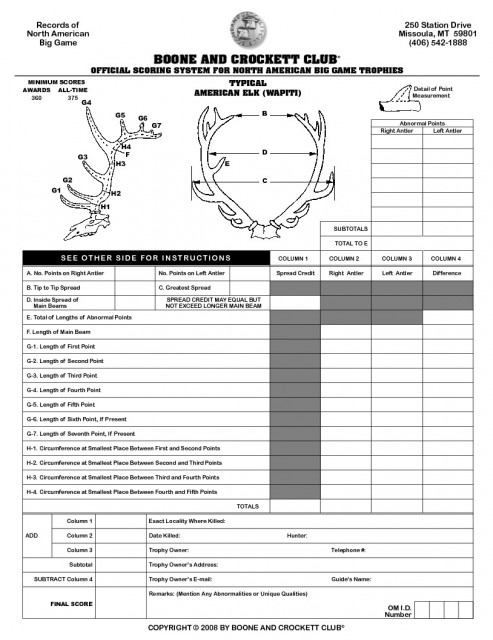In this section of the Scoring Feature, we’ll walk you through the Boone and Crockett (Pope & Young) Scoring method.To begin, you’ll need the following items:

Start by measuring the length of each tine on one side of the rack – if your bull is a 6X6, there will be 5 tines to measure (G1 thru G5). To accurately calculate the length of each tine, measure along the outside of the tine from the tip to the place where the tine intersects the nearest edge of the main beam (not the center of the main beam or the outside edge of the main beam). This intersection is best located by using your tape measure to locate the inside edge of the main beam and marking that “imaginary edge” with masking tape (see Figure 1 below).Record the point lengths in the appropriate boxes, measuring to the nearest 1/8 inch.

Next, record the length of the main beam. This measurement follows the outside contour of the beam from the tip of the beam to the lowest outside edge of the “burr”.

Circumference measurements of the main beam are then taken beginning between the 1st and 2nd tines (G1 and G2). A total of 4 measurements (H1 – H4) are recorded per side at the smallest point of circumference (see Figure 2) between the first four sets of tines.

If there are any “abnormal” points (i.e., points that originate from another tine or from the bottom or side of the main beam, or points that are not generally paired from one side of the rack to the other) add them up and record the total measurement of abnormal points.

Repeat the tine length, main beam, circumference, and abnormal point measurements for the other side of the rack.

Finally, measure the spread inside the main beams at the widest point and record this measurement.

For typical elk, adding these measurements together will determine the “gross” score of your bull. To determine the “net” score, you would calculate the differences between each measurement from the left side to the  right side and subtract the total deductions, as well as the total abnormal point lengths, from the “gross” score. For example, if the G1 on the left side was 16 3/8″ and the G1 on the right side was 14 1/8″, the total deduction for G1 would be 2 2/8″. If the bull is a 5X6 and has a 6 1/8″ G5 on the left with no G5 on the right, the entire 6 1/8″ G5 would be a deduction.

The other measurements indicated on the Boone and Crockett score sheet are simply for reference and are not used to calculate the final score.